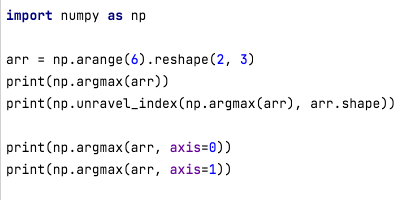# Python np.argmax() functionNumPy (np) is one of the most popular libraries for mathematical and scientific calculations. It provides a lot of functions to work with multidimensional arrays. In this article, we will focus on Python np.argmax() function.

## Python np.argmax() function

As the name suggests, the argmax() function returns the index of the maximum value in the NumPy array. If there are multiple indices with the same max values, the first index will be returned.

argmax() syntax:

np.argmax(aaxis=Noneout=None*keepdims=<no value>)

The first argument is the input array. If there is no axis provided, the array is flattened and then the index of max value is returned.

If we specify the axis, it returns the index value along the given axis.

The third argument is used to pass an array argument to store the result, it should be of the correct shape and data type to work properly.

If keepdims is passed as True, the axes which are reduced are left in the result as dimensions with size one.

Let’s look at some examples of using argmax() function to understand the usage of different arguments properly.

### 1. Find the index of maximum value using np.argmax()

```>>> import numpy as np
>>> arr = np.array([[4,2,3], [1,6,2]])
>>> arr
array([[4, 2, 3],
[1, 6, 2]])
>>> np.ndarray.flatten(arr)
array([4, 2, 3, 1, 6, 2])
>>> np.argmax(arr)
4
```

The np.argmax() returns 4 because the array is first flattened and then the index of max value is returned. So in this case, the max value is 6 and its index in the flattened array is 4.

But, we want the index value in a normal array, not the flattened one. So, we have to use the argmax() with the unravel_index() function to get the index value in the proper format.

```>>> np.unravel_index(np.argmax(arr), arr.shape)
(1, 1)
>>>
```

### 2. Finding the index of max value along an axis

If you want the index of max values along different axes, pass the axis parameter value. If we pass axis=0, the index of max values along the column is returned. For axis=1, the index of max values along the row is returned.

```>>> arr
array([[4, 2, 3],
[1, 6, 2]])
>>> np.argmax(arr, axis=0)
array([0, 1, 0])
>>> np.argmax(arr, axis=1)
array([0, 1])
```

For axis = 0, the first column values are 4 and 1. So the max value index is 0. Similarly, for the second column, the values are 2 and 6, so the max value index is 1. For the third column, values are 3 and 2, so the max value index is 0. That’s why we are getting the output as an array([0, 1, 0]).

For axis = 1, the first row values are (4, 2, 3), so the max value index is 0. For the second row, values are (1, 6, 2), so the max value index is 1. Hence the output array([0, 1]).

### 3. Using np.argmax() with multiple maximum values

```>>> import numpy as np
>>> arr = np.arange(6).reshape(2,3)
>>> arr
array([[0, 1, 2],
[3, 4, 5]])
>>> arr = 5
>>> arr
array([[0, 5, 2],
[3, 4, 5]])
>>> np.argmax(arr)
1
>>> arr = 5
>>> arr
array([[0, 5, 5],
[3, 4, 5]])
>>> np.argmax(arr)
1
>>> np.argmax(arr, axis=0)
array([1, 0, 0])
>>> np.argmax(arr, axis=1)
array([1, 2])
>>>
```

We are using arange() function to create a 2d array with some default values. Then we are changing one of the values to have multiple indexes with the max value. It’s clear from the output that the first index of the max value is returned when there are multiple places with the max value.

## Summary

NumPy argmax() function is easy to understand, just remember that the array is flattened before finding the index of the max value. Also, the axis argument is very helpful in finding the indices of the max values along rows and columns.